The problem to get a smooth transition between two curves appears very often. I had this problem a lot when working with simulations of DAE systems where some parameters were defined by empiric functions that did not fit well at the intersection point. An approach that is very easy to implement is the use of the tanh() function (hyperbolic tangent). This function is available in many of the modern programming languages.

Let’s exercise a simple example: f(x) = 1/x and  g(x) = x². Obviously both functions intersect at x=1. We want a function that follows f(x) left of the intersection and g(x) for values right of this point.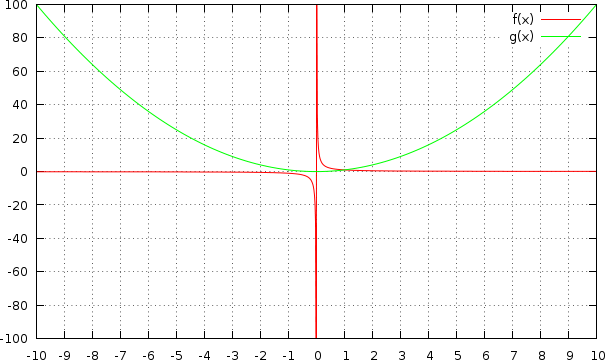To get a smooth transition at the intersection (x=1) we need a third function that smoothly “switches” between two values at a defined point. The pure tanh() function shows this behaviour, it returns values close to -1 for x<<0 and values close to 1 for x>>0. We define our new function s(x) = 0.5+0.5 tanh((x-a)/b). The function was shifted to return values between 0 and 1 and the parameters a and b can be used to define the switch point and the smoothing level. With a=1 (intersection point between f(x) and g(x)) and b=1 it shows the following curve: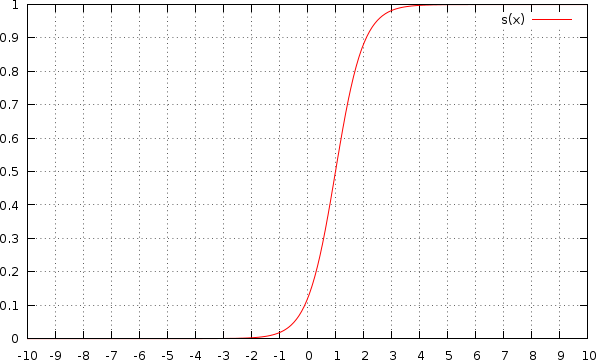With b=3 it is much smoother: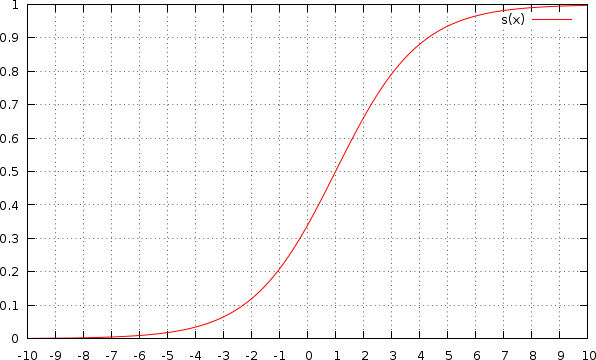And with b=0.1 it looks more like a step function: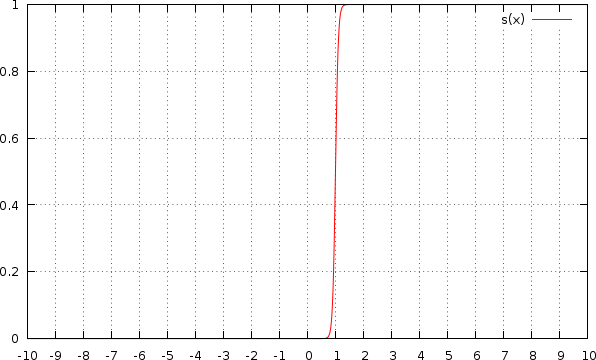In the last step we only need to combine the new switch function s(x) with the original functions: h(x) = s(x) g(x) + (1-s(x)) f(x). The result is a smooth curve that meets our requirements: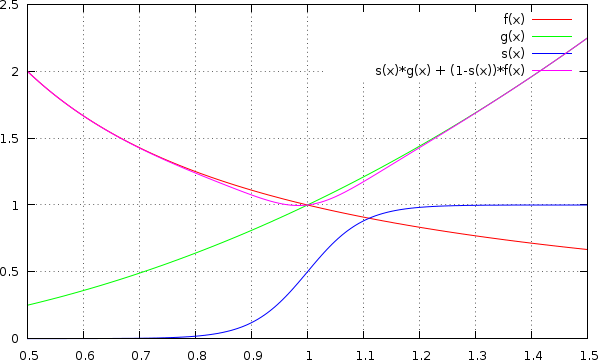The smoothing level can be adjusted with the parameter b. The example above uses b=0.1. The following chart shows the results with b=0.05: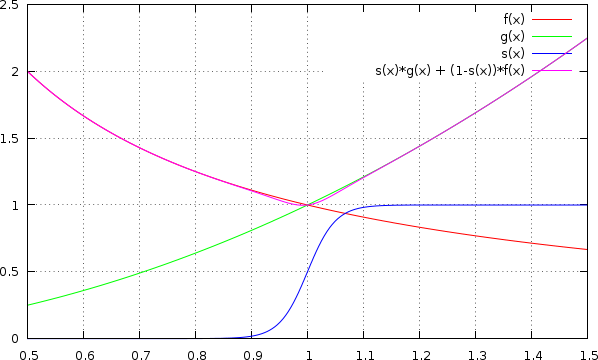This method works even at points where both functions  do not intersect. The smoothing function s(x) can fill the gap between f(x) and g(x). For the next chart the parameter a was changed from 1 to 1.2 (with b=0.01).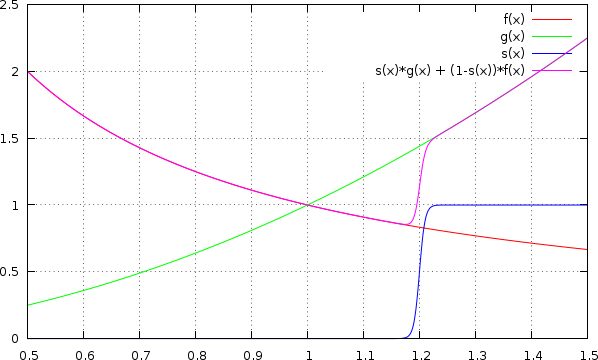The result may not be perfect for further calculations, but it is a smooth, continous function (given that f(x) and g(x) have the same quality). Since s(x) will never reach 0 or 1, the resulting function h(x) will differ from f(x) and g(x) over the whole range. This difference can be minimized with small values of b, but it will never disappear. If you can’t tolerate this, you need to replace s(x) with another function. One possibility is a piece of the sin()-function combined with a constant part, like the SinSteps interpolation method used in ncDataReader2.

You can play a bit with the example above using this gnuplot script. It reproduces all pictures shown in this post. In gnuplot press ENTER to continue.

```set samples 1000
set xtics 1

f(x) = 1/x
g(x) = x*x
plot f(x), g(x)
pause -1

s(x) = 0.5+0.5*tanh((x-a)/b)
a = 1
b = 1
plot s(x)
pause -1

b = 3
replot
pause -1

b = 0.1
replot
pause -1

set xrange [0.5:1.5]
set xtics 0.1

plot f(x), g(x), s(x), s(x)*g(x) + (1-s(x))*f(x)
pause -1

b = 0.05
replot
pause -1

a = 1.2
b = 0.01
replot
pause -1```